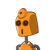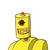# Can we say [ ( -24 ) ÷ 4 ] ÷ ( -2 ) = ( -24 ) ÷ [ 4 ÷ ( -2 ) ] ?​

Can we say [ ( -24 ) ÷ 4 ] ÷ ( -2 ) = ( -24 ) ÷ [ 4 ÷ ( -2 ) ] ?​

### 2 thoughts on “Can we say [ ( -24 ) ÷ 4 ] ÷ ( -2 ) = ( -24 ) ÷ [ 4 ÷ ( -2 ) ] ?​”

1.2.LHS is not equal to RHS

Step-by-step explanation:

[ ( -24 ) ÷ 4 ] ÷ ( -2 ) = ( -24 ) ÷ [ 4 ÷ ( -2 ) ]

( -6 ) ÷ (-2) = (-24) ÷ (-2)

3 = 12

LHS is not equal to RHS